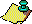Contents Next: Behavior of the Up: The 3x+1 problem. Previous: What is the

The results proved so far can be used to obtain an upper bound for the number of elements not having a finite stopping time. Let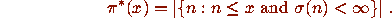The following result is the sharpest known result concerning the size of the ``exceptional'' set of n with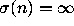.

### Theorem F

There is a positive constant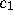such that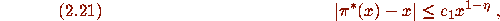where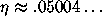is the constant defined in Theorem D.

### [Proof]Contents Next: Behavior of the Up: The 3x+1 problem. Previous: What is the